# Rosenfeld, Moshe

## Hamilton decomposition of prisms over 3-connected cubic planar graphs ★★

Author(s): Alspach; Rosenfeld

Conjecture   Every prism over a-connected cubic planar graph can be decomposed into two Hamilton cycles.

Keywords:

## Every prism over a 3-connected planar graph is hamiltonian. ★★

Author(s): Kaiser; Král; Rosenfeld; Ryjácek; Voss

Conjecture   Ifis a-connected planar graph, thenhas a Hamilton cycle.

Keywords:

## A gold-grabbing game ★★

Author(s): Rosenfeld

Setup Fix a treeand for every vertex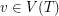a non-negative integer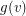which we think of as the amount of gold at.

2-Player game Players alternate turns. On each turn, a player chooses a leaf vertexof the tree, takes the gold at this vertex, and then deletes. The game ends when the tree is empty, and the winner is the player who has accumulated the most gold.

Problem   Find optimal strategies for the players.

Keywords: game; tree

## Coloring the Odd Distance Graph ★★★

Author(s): Rosenfeld

The Odd Distance Graph, denoted, is the graph with vertex setand two points adjacent if the distance between them is an odd integer.

Question   Is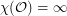?

Keywords: coloring; geometric graph; odd distance

## A generalization of Vizing's Theorem? ★★

Author(s): Rosenfeld

Conjecture   Letbe a simple-uniform hypergraph, and assume that every set of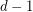points is contained in at mostedges. Then there exists an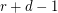-edge-coloring so that any two edges which sharevertices have distinct colors.

Keywords: edge-coloring; hypergraph; Vizing